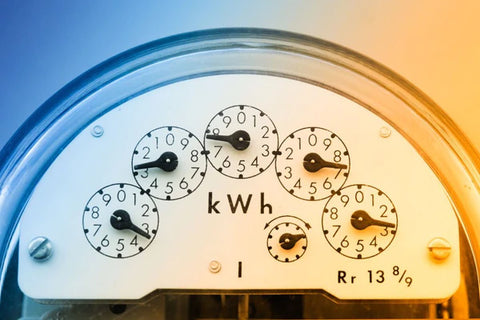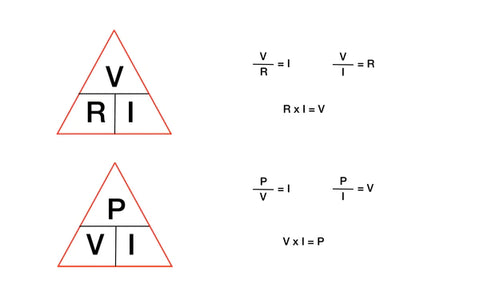Amps to Volts Calculator - ShopSolar.com

# Amps to Volts Calculator

In this amps-to-volts calculator, you’ll gain a better understanding of your electrical systems.

When it comes to calculating the difference from amps to volts, there is a simple mathematical equation that we can use to do the calculation.

To understand this formula, we need to understand Power (P), Voltage (V), and Current (I).

 Select calculation: amps to volts with watts amps to volts with ohms Enter amps: A Enter watts: W Enter ohms: Ω Result in volts: V

## Power, Voltage, and Current

Power is a measure of the rate at which current moves and we measure it in watts. You can think of power as the speed at which electrical energy travels through a circuit. It can also be referred to as wattage.You also need to understand voltage which is a form of pressure that forces charged electrons to move through the circuit. It is measured in volts and these are normally listed on the device.

Lastly, we have current, which is what is actually happening when electricity moves from one point to another. It is measured in amperes, which are commonly referred to as amps.

For smaller devices or batteries, you might want to convert amps to milliamps (mA)

Once we understand these three concepts, we can understand how they work together. Power is equal to the Voltage multiplied by the Current.

This means that the output is determined by the amount of current and the voltage at which that current is being moved through the circuit.

This formula can be written as P = V x I.

## Amps to Volts CalculatorNow that we have this formula, how do we use it to calculate amps to volts? In order to use the amps to volts calculator, you need to know both the power and the current.

In order to work out the volts, you need to take the Power (in watts) divided by the Current (in amps).

That looks like V = P/I.

We can look at the Bluetti AC200 Max power station as an example. This solar generator has a battery with a wattage of 2,048 watts and an Ah rating of 40.This Ah rating is connected to the current, but refers to amp hours, not amps. An amp hour is the amount of current that 1 amp can produce in 1 hour.

Depending on your needs, you might want to convert Ah to Wh to understand your energy consumption to determine the size of your solar system.

From this Ah rating, we can calculate the amps of the Bluetti AC200 by using an amp-hour calculator. 40 Ah means that the battery is capable of discharging 40 amps in 1 hour.

Using this, we can then say that V = 2048/40. This gives us a voltage of 51.2V, which is the voltage of this specific solar generator.

Sometimes you might need a more complex solution, like an amps, watts, & volts calculator to help you better understand the relationship between the units.

## Importance of Amps to Volts Calculator

When using electrical equipment, or choosing a complete solar panel kit, you need to be aware of the specifications of what you are working with.

You need to ensure that all the various parts of your system are compatible. For example, if you are choosing a battery to use along with your solar panels, you need to make sure that the voltages of each match up.

When it comes to complete solar systems, a good rule of thumb is to start with the voltage of your battery bank. You then use this to choose an inverter, and from there you choose your solar panels.

Make sure that you are using a common voltage. If you are unsure of the voltage, you can use the amps to volts calculator.

It is also important to remember to convert DC amps to AC amps when using solar power to run your home.

You can find all of the we have created in order to help you plan, size and install your own solar power system here.

## Join the Solar Lab!